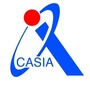IEEE神经网络和学习系统会刊TNNLS（IEEE Transactions on Neural Networks and learning systems）发表了关于神经网络和相关学习系统的理论、设计和应用的技术文章。本杂志的文章按照IEEE PSPB操作手册(章节8.2.1.C和8.2.2.A)的要求进行同行评审。每一篇发表的文章都由至少两名独立的审稿人通过单盲的同行评审过程进行评审，审稿人的身份作者并不知道，但审稿人知道作者的身份。文章在被接受前将筛选是否抄袭。 官网地址：http://dblp.uni-trier.de/db/journals/tnn/0+0+0+0+0+0+0+0+0+0+0+0+0+0+0+0+0+0+0+0+0+0+0+0+0+0+0+0+0+0+0+0+0+0+0+0+0+0+0+0+0+0+0+0+0+
Top